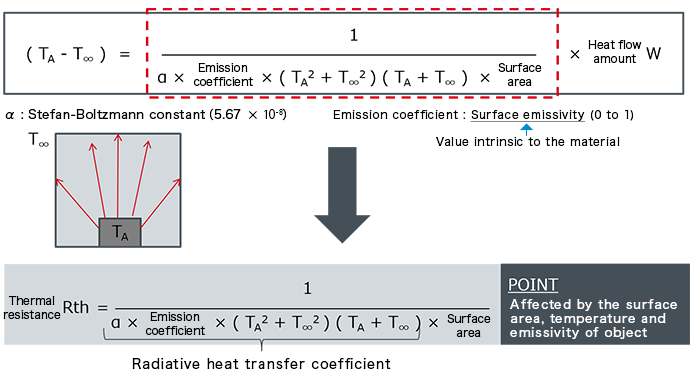# Fundamentals of Thermal Resistance and Heat Dissipation : Thermal Resistance in Emission

2021.04.21

・In thermal emission, heat movement occurs due to electromagnetic waves; the mechanism differs from those of conduction and convection, in which heat movement occurs by means of molecules.

・Thermal resistance in emission is the reciprocal of the product of the radiative heat transfer coefficient and the surface area of the heat-emitting body.

In succession to the heat transfer modes of conduction and convection, this article concerns thermal resistance in the third mode, emission.

Emission here means movement of heat by electromagnetic waves. This mechanism differs from those of conduction and convection, in which heat moves via molecules. Heat movement by emission is possible even in vacuum, where no objects or fluids are present.

### Thermal Resistance in Emission

Equations representing thermal resistance in emission are shown below.Thermal resistance in emission is the reciprocal of the product of the radiative heat transfer coefficient and the surface area of the heat-emitting body. From the equation, we see that the thermal resistance of emission is affected by the surface area, temperature, and emissivity of the object.

In this and the previous articles we have presented equations for thermal resistance in the three modes of heat transmission, which are conduction, convection, and emission. All are basic equations, and the relations between the terms in the equations and thermal resistance should be firmly understood.